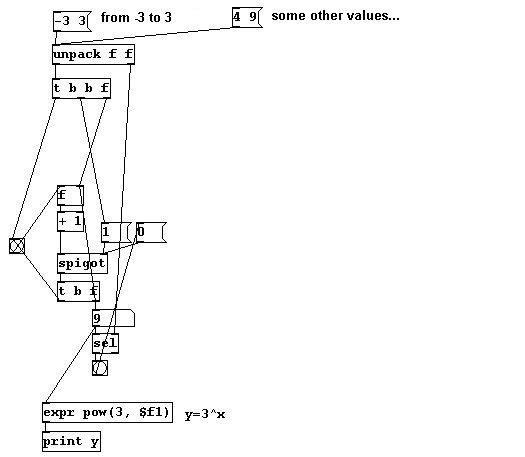## 2.2.2.2.6

a) A sequence of lists with pitch and volume:b) A function that, given a list of two numbers (the start and end values for the x-domain), can calculate the y-values - e.g., to calculate the values of the function y = 3x for the range from x = -2 to x = 4: